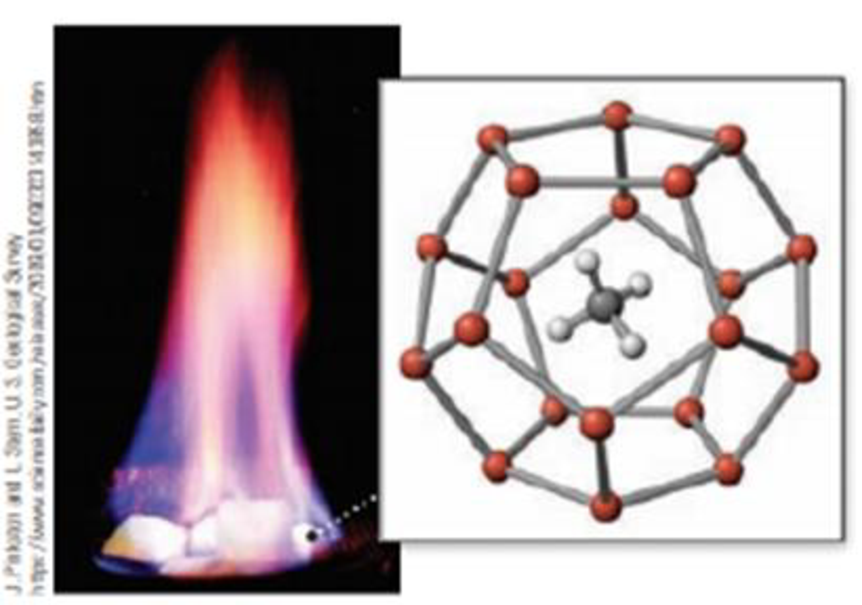Chapter 20, Problem 31PS

Chapter
Section
Textbook Problem

In methane hydrate the methane molecule is trapped in a cage of water molecules. Describe the structure: (a) how many water molecules make up the cage, (b) how many hydrogen bonds are involved, and (c) how many faces does the cage have? (Figure 20 16.)(a) Methane hydrate burns as methane gas escapes from the solid hydrate.(b) Methane hydrate consists of a lattice of water molecules with methane molecules trapped in the cavity.

(a)

Interpretation Introduction

Interpretation: The number of water molecules in the cage of methane hydrate has to be determined.

Concept introduction:

Methane hydrate: The structure of methane hydrate consists of having a methane molecule trapped in a cage of water molecules. It is considered to be a potential source of fuel in coming future.

At high pressure, if water is condensed in the occurrence of methane, methane hydrates or methane clathrates are formed.

Dodecahedron structure:

Number of faces is12

Number of vertices is 20

Number of diagonals is 160

Number of edges is 30

Explanation

Methane is trapped in the cage of water molecules in the compound methane hydrate.

The cage of water molecules is in dodecahedron structure...

(b)

Interpretation Introduction

Interpretation: The number of hydrogen bonds in the cage of methane hydrate has to be determined.

Concept introduction:

Methane hydrate: The structure of methane hydrate consists of having a methane molecule trapped in a cage of water molecules. It is considered to be a potential source of fuel in coming future.

At high pressure, if water is condensed in the occurrence of methane, methane hydrates or methane clathrates are formed.

Dodecahedron structure:

Number of faces is12

Number of vertices is 20

Number of diagonals is 160

Number of edges is 30

(c)

Interpretation Introduction

Interpretation: The number of faces in the cage of methane hydrate has to be determined.

Concept introduction:

Methane hydrate: The structure of methane hydrate consists of having a methane molecule trapped in a cage of water molecules. It is considered to be a potential source of fuel in coming future.

At high pressure, if water is condensed in the occurrence of methane, methane hydrates or methane clathrates are formed.

Dodecahedron structure:

Number of faces is12

Number of vertices is 20

Number of diagonals is 160

Number of edges is 30

Still sussing out bartleby?

Check out a sample textbook solution.

See a sample solution

The Solution to Your Study Problems

Bartleby provides explanations to thousands of textbook problems written by our experts, many with advanced degrees!

Get Started

A(n) always has the same composition.

Introductory Chemistry: A Foundation

6.25 Define the term ground state.

Chemistry for Engineering Students

Describe the relationship between epinephrine and fat mobilization.

Chemistry for Today: General, Organic, and Biochemistry